## Python Basics

Certification Training
3929 Learners
2 Chapters +

# Python Functions: Python Basics

This is the ‘Python Functions’ tutorial offered by Simplilearn. This tutorial is part of the 'Data Science with Python course' and we will get detailed information on various Python Functions.

## Objectives

After completing this Python functions tutorial you'll be able to

• Define a function and its advantages.
• Explain the procedures to create and call a function.
• Define recursion and its uses in programming.

## Introduction to Python Functions

A function is a block of organized, reasonable code used to perform a single and related action. The important benefits of functions are:

• Better modularity for applications
• Higher ability to reuse code

As we already know, Python gives us many built-in functions like print, but we can also create our own functions. These functions are called user-defined functions. To put it more formally, a function can be defined as a named sequence of statements that performs a computation. When we define a function, we specify the name and sequence of statements and later we can call the function by the given name. Let us look at the example below to understand how to call a function.

>>>type(10)

<type ‘int’>

This is an inbuilt function defined in the python library.

### Creating a Python Function

To understand how to create our own functions by using the Python programming language and how to use them later, refer to the syntax below

def func_name (arguments): statements

• The definition of a function starts with the given keyword.
• Then we have to provide a name for that function. We can pass some parameters to the function as well.
• Post that we need to write some statements that should execute whenever that function is called.

Arguments are optional. A function can have and cannot have arguments, and they can be in multiple numbers. Let us look at the example of a simple function below.

def hello_func():

Print “Hello from hello_func”

A simple function has been created with the name hello_func. No parameter has been passed to this function. Once this function is called, it will print “Hello from the given name”.

### Python Call Function

Defining a Python function only gives it a name, specifies the parameters that are to be included in the function and structures the blocks of a code. Once the basic structure of a function is finalized, we can execute it by calling it from

• another function or
• directly from the Python prompt

To call a function, we just need to type the name of that function, followed by round brackets. In the given example, the function: >>>hello_func(), will print the message ‘Hello’. If we have any list of arguments, it will be passed inside the brackets. The sequence of arguments passed while calling a function should be the same as it is in the function's definition. A function should be created before it's used in the program.

Look at the other elements of the Python Basic course here. Click to know more!

### Python Argument

There can be situations when we want to pass some information to a function. We can pass arguments to the function and use the ‘return’ statement. To understand this better let us look at the example below:

return x+y

A Python function has been created, which can add two numbers. If we want the function to send out any two numbers, add those numbers and send the result back to the user, then we would need to mention that the two variables x and y will be passed by the caller of that function. These two variables are shown within curly brackets

### Python Return Statement

Once we add these numbers using the plus operator, we can return the result to the caller of the function by using the keyword ‘return’ as shown in the function definition. The return statement will return that control to the caller, whenever called. If it's not followed by some value, it will still return the control to the caller, but will not return any value.

The return statement followed by some variable or value returns the value to the caller of the function. In case of the add function, we can return the addition of the result to the caller using the return statement. We can also set the default value of a variable in a function definition, by mentioning the variable name to be equal to the default value.

In the given example below:

return x+y

y=2 is set as the default value.

In any situation, if the caller calls this function with only one argument, then the default value of Y will be used.### Python Variable - Length Arguments

We may need to process a function for more arguments than what we specified while defining the function. These arguments are called variable-length arguments and are not named in the function definition.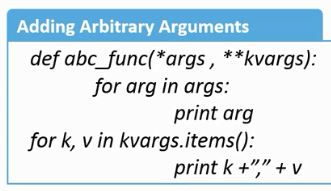An asterisk is placed before the variable name that holds the values of all non-keyword variable arguments. This remains empty if no additional arguments are specified during the function call. We can pass an arbitrary number of key-value pair arguments by using the asterix sign twice.

In the above example, the given keyword allows us to pass the variable-length of arguments to a function. We should use the given keyword if we want to handle the name arguments in a function.

Below is an example code to call this Python function.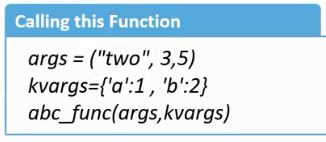Args is a list of unnamed of variables passed by a caller. The given function contains the list of arguments passed by a caller with the name.

Another example below shows how to create and call a function.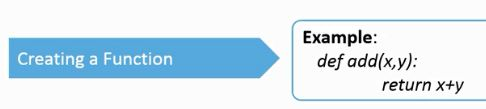In the given example, a function has been created, named add. This function accepts to arguments x and y. In the function definition, x and y are added and have returned the result using the return keyword. We can also call this function, by passing different argument values.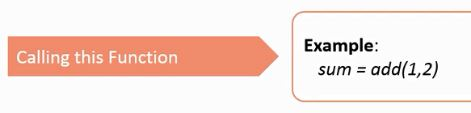In the above example, the add function has been called with values 1 and 2. This function will return 3 after addition.

Keen on learning more of Python Basics? Click to know more!

### Python Recursion

It is possible for one function to call another and it is also possible for a function to call itself. This is called Recursion. In literal terms, Recursion means defining something in terms of itself and is a very powerful feature. The given example below can help understand this better.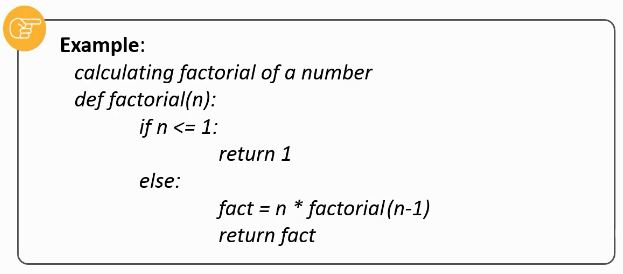• A Python function has been created which is calculating the factorial of a number. Now, suppose the caller of this function calls it with a value five.
• In the first statement, the function will check if the value is less than or equal to one.
• If yes, it returns one.
• Otherwise, it will multiply five with the factorial of four, which is, in turn, equal to the five factorial.

This demonstration below shows the steps to create a function in python.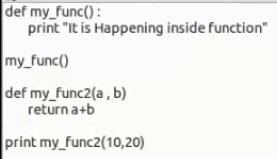• Create a new file called function_example.py
• Define a function called my_func()  using the given keyword.
• Write some ‘body’ in the function.
• We can call this function just by writing its name followed by brackets.
• Save it and run this code.
• Create a Python function which gets arguments as an input and returns some value.
• To call this function and pass values to it, write the name of the function and pass values in brackets.
• Save the code and run it.

## Key Takeaways

Let's summarize the topics covered in this lesson.

• A function is a block of organized, reusable code used to perform a single and related action.
• Once a function is defined, you can execute it by calling it from another function and the Python prompt.
• In situations where you need information for a function; pass arguments to functions.
• Recursion means defining something in its own terms. This function calls itself and is a way to repeat some action with different values.

## Conclusion

With this, we come to the end of the ‘Functions’ tutorial. The next tutorial is ‘Classes.’

Related Courses
Learner Reviews
• Disclaimer
• PMP, PMI, PMBOK, CAPM, PgMP, PfMP, ACP, PBA, RMP, SP, and OPM3 are registered marks of the Project Management Institute, Inc.

Name*
Email*
Phone Number*